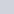``
`Please remember to cancel your appointment if you no longer need it. This can be done via the phone or online using Patient Access. Remembering to cancel your appointment means that another patient is able to be seen and will help with access. Thank you    There is now a free shuttle bus that runs from the new bus Interchange to the Town Hall. It runs roughly every 15 minutes from 8am (subject to change - Please click this text for more information and timetables)`

# `Welcome`

`City Walls Medical Centre is located in Chester, just a short walk from the town centre and university.`

`We also have a branch doctors surgery located in the village of Saughall, situated approximately 5 miles north west of Chester.`

`City Walls Medical Centre has been a training practice for student nurses since 2015. We have a supportive and knowledgeable nursing team that provide a positive learning environment for the students to achieve their potential and gain experience of working in Primary Care. `

`City Walls Medical Centre is a member of Chester South Primary Care Network (PCN). This is a group of GP practices that work together, share ideas and develop new ways of working to enable greater sustainability and improved patient care.`

Our Vision:

To make a positive difference in our patients’ lives through expert advice and personal empowerment.

`Practice News`

• `Community Pharmacy Opening Hours – Jubilee Bank Holiday 2022 May 30, 2022`
• `My Way Diabetes May 6, 2022`
• `Improving Veterans’ Mental and Physical Health Services in England April 26, 2022`
• `GP Average Earnings 2020-21 March 15, 2022`
• `Attending the Practice – Covid-19 February 25, 2022`

`BBC News – Health`

• `'I've been let down - I shouldn't have stage-4 cancer' August 10, 2022`
• `UK heatwave: Four-day extreme heat warning for parts of UK August 10, 2022`
• `Urgent polio boosters for London children August 10, 2022`
• `Polio: What is it and how does it spread? August 10, 2022`
• `Olivia Newton-John's cancer-research legacy August 9, 2022`

`NHS England`

• `NHS marks milestone in recovery plan as longest waits virtually eliminated August 8, 2022`
• `Heat-health watch alert: Level 3 – Heatwave action August 8, 2022`
• `Heat-health watch alert: level 2 – alert and readiness August 5, 2022`
• `Heat-Health Alert Level 1 – Summer preparedness August 4, 2022`
• `NHS to roll out life-changing glucose monitors to all Type 1 diabetes patients August 1, 2022`

### `ONLINE SERVICES`

` `
` Care Hub - Find Care Providers`
``
``

## `Facilities`

• `City Walls m/c`
• `saughall m/c`
` `

### `Disabled`

`5 Disabled Parking Spaces`

`Access Ramp`

`Passenger Lift`

` `

### `Car Park`

`There is a patient only car park available for use during visits to the practice only.`

` `

### `Health Hub`

`Free to use facilities to record blood pressure, BMI details and more.`

`About City Walls Medical Centre (click to view)`
``` /* */ var ThriveApp = {"ajax_url":"https:\/\/www.citywallsmedicalcentre.co.uk\/wp-admin\/admin-ajax.php","lazy_load_comments":"1","comments_loaded":"0","theme_uri":"https:\/\/www.citywallsmedicalcentre.co.uk\/wp-content\/themes\/focusblog","translations":{"ProductDetails":"Product Details"}}; /* */ /* */ var tve_dash_front = {"ajaxurl":"https:\/\/www.citywallsmedicalcentre.co.uk\/wp-admin\/admin-ajax.php","force_ajax_send":"1","is_crawler":"","recaptcha":[],"post_id":"4"}; /* */ /* */ var q2w3_sidebar_options = [{"sidebar":"sidebar-1","use_sticky_position":true,"margin_top":100,"margin_bottom":0,"stop_elements_selectors":"footer","screen_max_width":940,"screen_max_height":0,"widgets":["#nav_menu-3"]},{"sidebar":"sidebar-2","use_sticky_position":true,"margin_top":100,"margin_bottom":0,"stop_elements_selectors":"footer","screen_max_width":940,"screen_max_height":0,"widgets":["#nav_menu-2"]}]; /* */ /* */ var wpcf7_recaptcha = {"sitekey":"6LcjLIMUAAAAACyJ-zhx4F5hPrKb1W0LITQcy2vW","actions":{"homepage":"homepage","contactform":"contactform"}}; /* */ var tcb_post_lists=JSON.parse('[]');/**/if ( !window.TL_Const ) {var TL_Const={"security":"22da65c551","ajax_url":"https:\/\/www.citywallsmedicalcentre.co.uk\/wp-admin\/admin-ajax.php","forms":[],"action_conversion":"tve_leads_ajax_conversion","action_impression":"tve_leads_ajax_impression","ajax_load":1,"main_group_id":2096,"display_options":{"allowed_post_types":[],"flag_url_match":null},"custom_post_data":{"http_referrer":"https:\/\/www.citywallsmedicalcentre.co.uk\/chronic-disease-annual-review-invite-system\/"},"current_screen":{"screen_type":1,"screen_id":0},"ignored_fields":["email","_captcha_size","_captcha_theme","_captcha_type","_submit_option","_use_captcha","g-recaptcha-response","__tcb_lg_fc","__tcb_lg_msg","_state","_form_type","_error_message_option","_back_url","_submit_option","url","_asset_group","_asset_option","mailchimp_optin","tcb_token","tve_labels","tve_mapping","_api_custom_fields","_sendParams","_autofill"]};} else { window.TL_Front && TL_Front.extendConst && TL_Front.extendConst({"security":"22da65c551","ajax_url":"https:\/\/www.citywallsmedicalcentre.co.uk\/wp-admin\/admin-ajax.php","forms":[],"action_conversion":"tve_leads_ajax_conversion","action_impression":"tve_leads_ajax_impression","ajax_load":1,"main_group_id":2096,"display_options":{"allowed_post_types":[],"flag_url_match":null},"custom_post_data":{"http_referrer":"https:\/\/www.citywallsmedicalcentre.co.uk\/chronic-disease-annual-review-invite-system\/"},"current_screen":{"screen_type":1,"screen_id":0},"ignored_fields":["email","_captcha_size","_captcha_theme","_captcha_type","_submit_option","_use_captcha","g-recaptcha-response","__tcb_lg_fc","__tcb_lg_msg","_state","_form_type","_error_message_option","_back_url","_submit_option","url","_asset_group","_asset_option","mailchimp_optin","tcb_token","tve_labels","tve_mapping","_api_custom_fields","_sendParams","_autofill"]})} /* */ _stq = window._stq || []; _stq.push([ 'view', {v:'ext',j:'1:11.2',blog:'118849571',post:'4',tz:'1',srv:'www.citywallsmedicalcentre.co.uk'} ]); _stq.push([ 'clickTrackerInit', '118849571', '4' ]); !function(t,e){"object"==typeof exports&&"undefined"!=typeof module?module.exports=e():"function"==typeof define&&define.amd?define(e):(t="undefined"!=typeof globalThis?globalThis:t||self).LazyLoad=e()}(this,function(){"use strict";function e(){return(e=Object.assign||function(t){for(var e=1;e<arguments.length;e++){var n,a=arguments[e];for(n in a)Object.prototype.hasOwnProperty.call(a,n)&&(t[n]=a[n])}return t}).apply(this,arguments)}function i(t){return e({},it,t)}function o(t,e){var n,a="LazyLoad::Initialized",i=new t(e);try{n=new CustomEvent(a,{detail:{instance:i}})}catch(t){(n=document.createEvent("CustomEvent")).initCustomEvent(a,!1,!1,{instance:i})}window.dispatchEvent(n)}function l(t,e){return t.getAttribute(gt+e)}function c(t){return l(t,bt)}function s(t,e){return function(t,e,n){e=gt+e;null!==n?t.setAttribute(e,n):t.removeAttribute(e)}(t,bt,e)}function r(t){return s(t,null),0}function u(t){return null===c(t)}function d(t){return c(t)===vt}function f(t,e,n,a){t&&(void 0===a?void 0===n?t(e):t(e,n):t(e,n,a))}function _(t,e){nt?t.classList.add(e):t.className+=(t.className?" ":"")+e}function v(t,e){nt?t.classList.remove(e):t.className=t.className.replace(new RegExp("(^|\\s+)"+e+"(\\s+|\$)")," ").replace(/^\s+/,"").replace(/\s+\$/,"")}function g(t){return t.llTempImage}function b(t,e){!e||(e=e._observer)&&e.unobserve(t)}function p(t,e){t&&(t.loadingCount+=e)}function h(t,e){t&&(t.toLoadCount=e)}function n(t){for(var e,n=[],a=0;e=t.children[a];a+=1)"SOURCE"===e.tagName&&n.push(e);return n}function m(t,e){(t=t.parentNode)&&"PICTURE"===t.tagName&&n(t).forEach(e)}function a(t,e){n(t).forEach(e)}function E(t){return!!t[st]}function I(t){return t[st]}function y(t){return delete t[st]}function A(e,t){var n;E(e)||(n={},t.forEach(function(t){n[t]=e.getAttribute(t)}),e[st]=n)}function k(a,t){var i;E(a)&&(i=I(a),t.forEach(function(t){var e,n;e=a,(t=i[n=t])?e.setAttribute(n,t):e.removeAttribute(n)}))}function L(t,e,n){_(t,e.class_loading),s(t,ut),n&&(p(n,1),f(e.callback_loading,t,n))}function w(t,e,n){n&&t.setAttribute(e,n)}function x(t,e){w(t,ct,l(t,e.data_sizes)),w(t,rt,l(t,e.data_srcset)),w(t,ot,l(t,e.data_src))}function O(t,e,n){var a=l(t,e.data_bg_multi),i=l(t,e.data_bg_multi_hidpi);(a=at&&i?i:a)&&(t.style.backgroundImage=a,n=n,_(t=t,(e=e).class_applied),s(t,ft),n&&(e.unobserve_completed&&b(t,e),f(e.callback_applied,t,n)))}function N(t,e){!e||0<e.loadingCount||0<e.toLoadCount||f(t.callback_finish,e)}function C(t,e,n){t.addEventListener(e,n),t.llEvLisnrs[e]=n}function M(t){return!!t.llEvLisnrs}function z(t){if(M(t)){var e,n,a=t.llEvLisnrs;for(e in a){var i=a[e];n=e,i=i,t.removeEventListener(n,i)}delete t.llEvLisnrs}}function R(t,e,n){var a;delete t.llTempImage,p(n,-1),(a=n)&&--a.toLoadCount,v(t,e.class_loading),e.unobserve_completed&&b(t,n)}function T(o,r,c){var l=g(o)||o;M(l)||function(t,e,n){M(t)||(t.llEvLisnrs={});var a="VIDEO"===t.tagName?"loadeddata":"load";C(t,a,e),C(t,"error",n)}(l,function(t){var e,n,a,i;n=r,a=c,i=d(e=o),R(e,n,a),_(e,n.class_loaded),s(e,dt),f(n.callback_loaded,e,a),i||N(n,a),z(l)},function(t){var e,n,a,i;n=r,a=c,i=d(e=o),R(e,n,a),_(e,n.class_error),s(e,_t),f(n.callback_error,e,a),i||N(n,a),z(l)})}function G(t,e,n){var a,i,o,r,c;t.llTempImage=document.createElement("IMG"),T(t,e,n),E(c=t)||(c[st]={backgroundImage:c.style.backgroundImage}),o=n,r=l(a=t,(i=e).data_bg),c=l(a,i.data_bg_hidpi),(r=at&&c?c:r)&&(a.style.backgroundImage='url("'.concat(r,'")'),g(a).setAttribute(ot,r),L(a,i,o)),O(t,e,n)}function D(t,e,n){var a;T(t,e,n),a=e,e=n,(t=It[(n=t).tagName])&&(t(n,a),L(n,a,e))}function V(t,e,n){var a;a=t,(-1<yt.indexOf(a.tagName)?D:G)(t,e,n)}function F(t,e,n){var a;t.setAttribute("loading","lazy"),T(t,e,n),a=e,(e=It[(n=t).tagName])&&e(n,a),s(t,vt)}function j(t){t.removeAttribute(ot),t.removeAttribute(rt),t.removeAttribute(ct)}function P(t){m(t,function(t){k(t,Et)}),k(t,Et)}function S(t){var e;(e=At[t.tagName])?e(t):E(e=t)&&(t=I(e),e.style.backgroundImage=t.backgroundImage)}function U(t,e){var n;S(t),n=e,u(e=t)||d(e)||(v(e,n.class_entered),v(e,n.class_exited),v(e,n.class_applied),v(e,n.class_loading),v(e,n.class_loaded),v(e,n.class_error)),r(t),y(t)}function \$(t,e,n,a){var i;n.cancel_on_exit&&(c(t)!==ut||"IMG"===t.tagName&&(z(t),m(i=t,function(t){j(t)}),j(i),P(t),v(t,n.class_loading),p(a,-1),r(t),f(n.callback_cancel,t,e,a)))}function q(t,e,n,a){var i,o,r=(o=t,0<=pt.indexOf(c(o)));s(t,"entered"),_(t,n.class_entered),v(t,n.class_exited),i=t,o=a,n.unobserve_entered&&b(i,o),f(n.callback_enter,t,e,a),r||V(t,n,a)}function H(t){return t.use_native&&"loading"in HTMLImageElement.prototype}function B(t,i,o){t.forEach(function(t){return(a=t).isIntersecting||0<a.intersectionRatio?q(t.target,t,i,o):(e=t.target,n=t,a=i,t=o,void(u(e)||(_(e,a.class_exited),\$(e,n,a,t),f(a.callback_exit,e,n,t))));var e,n,a})}function J(e,n){var t;et&&!H(e)&&(n._observer=new IntersectionObserver(function(t){B(t,e,n)},{root:(t=e).container===document?null:t.container,rootMargin:t.thresholds||t.threshold+"px"}))}function K(t){return Array.prototype.slice.call(t)}function Q(t){return t.container.querySelectorAll(t.elements_selector)}function W(t){return c(t)===_t}function X(t,e){return e=t||Q(e),K(e).filter(u)}function Y(e,t){var n;(n=Q(e),K(n).filter(W)).forEach(function(t){v(t,e.class_error),r(t)}),t.update()}function t(t,e){var n,a,t=i(t);this._settings=t,this.loadingCount=0,J(t,this),n=t,a=this,Z&&window.addEventListener("online",function(){Y(n,a)}),this.update(e)}var Z="undefined"!=typeof window,tt=Z&&!("onscroll"in window)||"undefined"!=typeof navigator&&/(gle|ing|ro)bot|crawl|spider/i.test(navigator.userAgent),et=Z&&"IntersectionObserver"in window,nt=Z&&"classList"in document.createElement("p"),at=Z&&1<window.devicePixelRatio,it={elements_selector:".lazy",container:tt||Z?document:null,threshold:300,thresholds:null,data_src:"src",data_srcset:"srcset",data_sizes:"sizes",data_bg:"bg",data_bg_hidpi:"bg-hidpi",data_bg_multi:"bg-multi",data_bg_multi_hidpi:"bg-multi-hidpi",data_poster:"poster",class_applied:"applied",class_loading:"litespeed-loading",class_loaded:"litespeed-loaded",class_error:"error",class_entered:"entered",class_exited:"exited",unobserve_completed:!0,unobserve_entered:!1,cancel_on_exit:!0,callback_enter:null,callback_exit:null,callback_applied:null,callback_loading:null,callback_loaded:null,callback_error:null,callback_finish:null,callback_cancel:null,use_native:!1},ot="src",rt="srcset",ct="sizes",lt="poster",st="llOriginalAttrs",ut="loading",dt="loaded",ft="applied",_t="error",vt="native",gt="data-",bt="ll-status",pt=[ut,dt,ft,_t],ht=[ot],mt=[ot,lt],Et=[ot,rt,ct],It={IMG:function(t,e){m(t,function(t){A(t,Et),x(t,e)}),A(t,Et),x(t,e)},IFRAME:function(t,e){A(t,ht),w(t,ot,l(t,e.data_src))},VIDEO:function(t,e){a(t,function(t){A(t,ht),w(t,ot,l(t,e.data_src))}),A(t,mt),w(t,lt,l(t,e.data_poster)),w(t,ot,l(t,e.data_src)),t.load()}},yt=["IMG","IFRAME","VIDEO"],At={IMG:P,IFRAME:function(t){k(t,ht)},VIDEO:function(t){a(t,function(t){k(t,ht)}),k(t,mt),t.load()}},kt=["IMG","IFRAME","VIDEO"];return t.prototype={update:function(t){var e,n,a,i=this._settings,o=X(t,i);{if(h(this,o.length),!tt&&et)return H(i)?(e=i,n=this,o.forEach(function(t){-1!==kt.indexOf(t.tagName)&&F(t,e,n)}),void h(n,0)):(t=this._observer,i=o,t.disconnect(),a=t,void i.forEach(function(t){a.observe(t)}));this.loadAll(o)}},destroy:function(){this._observer&&this._observer.disconnect(),Q(this._settings).forEach(function(t){y(t)}),delete this._observer,delete this._settings,delete this.loadingCount,delete this.toLoadCount},loadAll:function(t){var e=this,n=this._settings;X(t,n).forEach(function(t){b(t,e),V(t,n,e)})},restoreAll:function(){var e=this._settings;Q(e).forEach(function(t){U(t,e)})}},t.load=function(t,e){e=i(e);V(t,e)},t.resetStatus=function(t){r(t)},Z&&function(t,e){if(e)if(e.length)for(var n,a=0;n=e[a];a+=1)o(t,n);else o(t,e)}(t,window.lazyLoadOptions),t});!function(e,t){"use strict";function a(){t.body.classList.add("litespeed_lazyloaded")}function n(){console.log("[LiteSpeed] Start Lazy Load Images"),d=new LazyLoad({elements_selector:"[data-lazyloaded]",callback_finish:a}),o=function(){d.update()},e.MutationObserver&&new MutationObserver(o).observe(t.documentElement,{childList:!0,subtree:!0,attributes:!0})}var d,o;e.addEventListener?e.addEventListener("load",n,!1):e.attachEvent("onload",n)}(window,document); ```